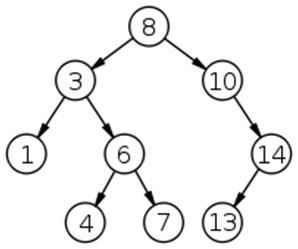Q:

# K-th smallest element in a Binary Search Tree

Find the k-th smallest element in a given binary search tree (BST).

Example:```    K=4
Kth smallest element in the above binary tree is: 6

K=5
Kth smallest element in the above binary tree is: 7```

One possible solution is to store the in-order traversal of the BST and printing the Kth element from the list. This can be done because in-order traversal of the BST produces a sorted list. But this solution leads to the overhead of additional storage which may not be entertained.

So, we go for a much better solution where we don’t need any additional space.

Algorithm:

```//k, count both parameter are passed by reference
FUNCTION kthSmallest (root, int & k, int &count)
1.  Base case
IF (root is NULL)
Return 0;
2. Carry out in-order traversal and keep checking for kth smallest element.
left=kthSmallest (root->left, k, count) //for left subtree
IF (left)
return left;
Increment count
IF (count==k)
return root->data; //kth smallest element

kthSmallest(root->right,k,count); //for right subtree

In the main function we call kthSmallest (root, k, 0) //count 0 initially
```

Example with explanation:```    In the main function we make call to kthSmallest(root, 4, 0)
-------------------------------------------------------------

KthSmallest (8, 4, 0)
8 not NULL
Left= KthSmallest(8->left , 4, 0);
Call to KthSmallest(3 , 4, 0): //8->left=3
-------------------------------------------------------------

KthSmallest (3, 4, 0)
3 not NULL
Left=KthSmallest(3->left , 4, 0);
Call to KthSmallest(1 , 4, 0): //3->left=1
-------------------------------------------------------------

KthSmallest (1, 4, 0)
1 not NULL
Left= KthSmallest(1->left , 4, 0);
Call to KthSmallest(NULL , 4, 0): //1->left=NULL
-------------------------------------------------------------

KthSmallest (NULL, 4, 0)
node is NULL
return 0;
-------------------------------------------------------------

Control back to KthSmallest (1, 4, 0):
Left=0
Increment count
Count=1;
K!=count
Call to kthSmallest(1->right, 4, 1)
-------------------------------------------------------------

This is again NULL and control backs to KthSmallest (1, 4, 0)
Since end of function reached control backs to KthSmallest (3, 4, 0)
KthSmallest (3, 4, 0):
Increment count
Count=2 //it’s not 1 because count is passed by reference
K!=count
Call to KthSmallest(3->right, 4, 2)
```

So if you carry out similar way you will be able to find the k-th smallest one once k==count

C++ implementation:

``````#include <bits/stdc++.h>
using namespace std;

// tree node is defined
class Node{
public:
int data;
Node *left;
Node *right;
};

// creating new node
Node* newnode(int data)
{
Node* node = (Node*)malloc(sizeof(Node));
node->data = data;
node->left = NULL;
node->right = NULL;
return(node);
}

//finding kth smallest element
int kthSmallest(Node *root, int& k,int &count){
if(!root)
return 0;

int left=kthSmallest(root->left,k,count);
if(left)
return left;
count=count+1;
if(count==k)
return root->data;

kthSmallest(root->right,k,count);
}

int main()
{
//building the bst
int count=0,k;

Node *root=newnode(8);

root->left= newnode(3);
root->right= newnode(10);
root->right->right=newnode(14);
root->right->right->left=newnode(13);
root->left->left=newnode(1);
root->left->right=newnode(6);
root->left->right->left=newnode(4);
root->left->right->right=newnode(7);

cout<<"input k\n";
cin>>k;

cout<<"Kth smallest element in the ";
cout<<"binary tree is :"<<endl;
cout<< kthSmallest(root,k,count);

return 0;
}
``````

Output

```input k
4
Kth smallest element in the binary tree is :
6```